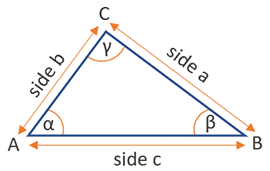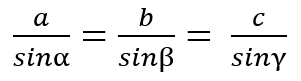# Perimeter of a Triangle Calculator

Use this calculator to easily calculate the perimeter of a triangle by the different possible pieces of information.

Share calculator:

Embed this tool:
get code

## Perimeter of a triangle formula

The formula for the perimeter of a triangle T is T = side a + side b + side c, as seen in the figure below:However, given different sets of other values about a triangle, it is possible to calculate the perimeter in other ways. These ways have names and abbreviations assigned based on what elements of the triangle they include: SSS, SAS, SSA, AAS and are all supported by our perimeter of a triangle calculator.

## Rules for solving a triangle

So, how to calculate the perimeter of a triangle using more advanced rules? As mentioned above, there are several different sets of measurements you can start with, from which you can solve the whole triangle, meaning you can arrive at the length of its sides as well.

• SSS (side-side-side) - this is the simplest one in which you basically have all three sides. Just sum them up according to the formula above, and you are done.
• SAS (side-angle-side) - having the lengths of two sides and the included angle (the angle between the two), you can calculate the remaining angles and sides, then use the SSS rule.
• SSA (side-side-angle) - having the lengths of two sides and a non-included angle (an angle that is not between the two), you can solve the triangle as well.
• ASA (angle-side-angle) - having the measurements of two angles and the side which serves as an arm for both (is between them), you can again solve the triangle fully.

Many of the above rules rely on the Law of Sines and the Law of Cosines, so if you are not familiar with them, it might be a bit tricky to understand them. The law of sines basically states that each side and its opposing angle's sine are related in the same way:The law of cosines is a generalization of the Pythagorean theorem and states that c2 = a2 + b2 - 2ab·cosγ using the notation from our calculator graph.

Another rule, supported by our perimeter of a triangle calculator is for right-angled triangles only: in such a triangle, if you are given the length of the hypotenuse and one of the other sides, you can easily compute the perimeter using the Pythagorean theorem.

## Examples: find the perimeter of a triangle

Example 1: In the simplest scenario one has measured all three sides of a triangle and then it is a matter of simple summation to find the perimeter. For example, if the sides are 3 in, 4 in, and 5 in, then the perimeter is simply 3 + 4 + 5 = 12 inches in total.

Example 2: In a slightly more complicated task, we are given two of the sides and the angle between them. This is then a straightforward application of the SAS rule by replacing the respective values. If sides b and c are equal at 6 feet and the angle is 30° then the length of side a is √b2 + c2 - 2 x b x c x cosα = √36 + 36 - 2 x 6 x 6 x cos(30°) = √72 - 72 x 0.866025 = √72 - 62.3538 = √9.65 = 3.1 ft. The perimeter is then 3.1 + 6 + 6 = 15.1 feet.

#### Cite this calculator & page

If you'd like to cite this online calculator resource and information as provided on the page, you can use the following citation:
Georgiev G.Z., "Perimeter of a Triangle Calculator", [online] Available at: https://www.gigacalculator.com/calculators/perimeter-of-triangle-calculator.php URL [Accessed Date: 01 Apr, 2023].